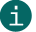# Check digit calculation of Modulo 10

Modulo 10

## This is a description of calculating check digits according to Modulo 10.ActiveBarcode calculates this check digits for most codes automatically.
This documentation is only for the sake of completeness.

Calculation of a checksum according to Modulo 10:
A check digit according to Modulo 10 is used by EAN codes, e.g. EAN-13.

With the EAN codes the check digit is calculated according to the modulo 10 calculation method with the weighting 3. Weighting 3 starts at the first digit from the right. Then the individual digits are multiplied by the weighting. These results are added to a sum. The modulo 10 is calculated from this sum. First the sum is divided by 10. The remainder of the division is subtracted from 10. The result of this subtraction is the checksum/check digit.

The following is a calculation example for Modulo 10 using an EAN-13 code:

 Digits: 4 0 0 7 6 3 0 0 0 0 1 1 Weight: 1 3 1 3 1 3 1 3 1 3 1 3 Results: 4+0+0+21+6+9+0+0+0+0+1+3 = 44 Calculate checksum: The addition to the next multiple of 10. (50) Check digit 6

With the Code 25 Codes, the check digit is also calculated with weighting 3 according to the Modulo 10 calculation method. However, weighting 3 starts at the first useful digit from the left. Then the individual useful digits are multiplied by the weighting. These resulting results are added to a sum. The modulo 10 is calculated from this sum. First the sum is divided by 10. The remainder of the division is subtracted from 10 (calculate the difference to 10). The result of this subtraction is the checksum/check digit.

In the following you can see a calculation example for Modulo 10 using Code 25:

 Digits: 1 2 3 4 5 Weight: 3 1 3 1 3 Results: 3+2+9+4+15 = 33 Calculate checksum: The addition to the next multiple of 10. (40) Check digit 7

With the Leitcode and the Identcode the check digit is also calculated according to the modulo 10 calculation method. However, the weighting is different here: Weighting of 4 for odd positions and 9 for straight positions. Here is an example:

 Digits: 2 3 6 6 9 0 1 2 0 1 2 3 0 Weight: 4 9 4 9 4 9 4 9 4 9 4 9 4 Results: 8+27+24+54+36+0+4+18+0+9+8+27+0 = 215 Calculate checksum: The addition to the next multiple of 10. (220) Check digit 5

A calculation example for Modulo 10 using an EAN-14 / ITF-14 / SCC-14 / DUN-14 code:

 Digits: 0 4 0 0 7 6 3 0 0 0 0 1 1 Weight: 3 1 3 1 3 1 3 1 3 1 3 1 3 Results: 0+4+0+0+21+6+9+0+0+0+0+1+3 = 44 Calculate checksum: The addition to the next multiple of 10. (50) Check digit 6